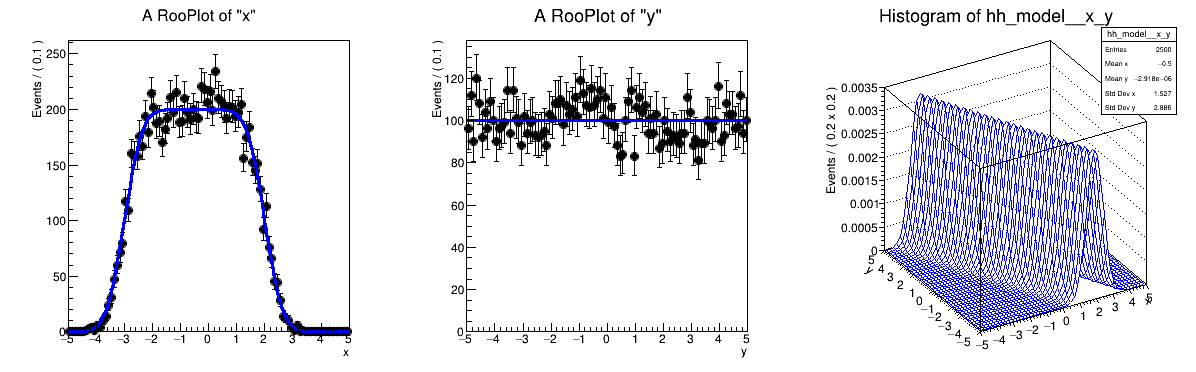# Rf 3 0 1_Composition¶

Multidimensional models: multi-dimensional pdfs through composition, e.g. substituting a pdf parameter with a function that depends on other observables

pdf = gauss(x,f(y),s) with f(y) = a0 + a1*y

Author: Clemens Lange, Wouter Verkerke (C++ version)
This notebook tutorial was automatically generated with ROOTBOOK-izer from the macro found in the ROOT repository on Saturday, November 28, 2020 at 10:44 AM.

In :
import ROOT

Welcome to JupyROOT 6.23/01


## Setup composed model gauss(x, m(y), s)¶

Create observables

In :
x = ROOT.RooRealVar("x", "x", -5, 5)
y = ROOT.RooRealVar("y", "y", -5, 5)

RooFit v3.60 -- Developed by Wouter Verkerke and David Kirkby
Copyright (C) 2000-2013 NIKHEF, University of California & Stanford University



Create function f(y) = a0 + a1*y

In :
a0 = ROOT.RooRealVar("a0", "a0", -0.5, -5, 5)
a1 = ROOT.RooRealVar("a1", "a1", -0.5, -1, 1)
fy = ROOT.RooPolyVar("fy", "fy", y, ROOT.RooArgList(a0, a1))


Creat gauss(x,f(y),s)

In :
sigma = ROOT.RooRealVar("sigma", "width of gaussian", 0.5)
model = ROOT.RooGaussian(
"model", "Gaussian with shifting mean", x, fy, sigma)

[#0] WARNING:InputArguments -- The parameter 'sigma' with range [-1e+30, 1e+30] of the RooGaussian 'model' exceeds the safe range of (0, inf). Advise to limit its range.


## Sample data, plot data and pdf on x and y¶

Generate 10000 events in x and y from model

In :
data = model.generate(ROOT.RooArgSet(x, y), 10000)

[#1] INFO:NumericIntegration -- RooRealIntegral::init(model_Int[x,y]) using numeric integrator RooIntegrator1D to calculate Int(y)
[#1] INFO:NumericIntegration -- RooRealIntegral::init(model_Int[x,y]) using numeric integrator RooIntegrator1D to calculate Int(y)
[#1] INFO:NumericIntegration -- RooRealIntegral::init(model_Int[x,y]) using numeric integrator RooIntegrator1D to calculate Int(y)


Plot x distribution of data and projection of model x = Int(dy) model(x,y)

In :
xframe = x.frame()
data.plotOn(xframe)
model.plotOn(xframe)

Out:
<cppyy.gbl.RooPlot object at 0x70c1560>
[#1] INFO:Plotting -- RooAbsReal::plotOn(model) plot on x integrates over variables (y)
[#1] INFO:NumericIntegration -- RooRealIntegral::init(model_Int[x,y]) using numeric integrator RooIntegrator1D to calculate Int(y)
[#1] INFO:NumericIntegration -- RooRealIntegral::init(model_Int[y]_Norm[x,y]) using numeric integrator RooIntegrator1D to calculate Int(y)


Plot x distribution of data and projection of model y = Int(dx) model(x,y)

In :
yframe = y.frame()
data.plotOn(yframe)
model.plotOn(yframe)

Out:
<cppyy.gbl.RooPlot object at 0x719d4f0>
[#1] INFO:Plotting -- RooAbsReal::plotOn(model) plot on y integrates over variables (x)
[#1] INFO:NumericIntegration -- RooRealIntegral::init(model_Int[x,y]) using numeric integrator RooIntegrator1D to calculate Int(y)


Make two-dimensional plot in x vs y

In :
hh_model = model.createHistogram("hh_model", x, ROOT.RooFit.Binning(
50), ROOT.RooFit.YVar(y, ROOT.RooFit.Binning(50)))
hh_model.SetLineColor(ROOT.kBlue)

[#1] INFO:NumericIntegration -- RooRealIntegral::init(model_Int[x,y]) using numeric integrator RooIntegrator1D to calculate Int(y)


Make canvas and draw ROOT.RooPlots

In :
c = ROOT.TCanvas("rf301_composition", "rf301_composition", 1200, 400)
c.Divide(3)
c.cd(1)
xframe.GetYaxis().SetTitleOffset(1.4)
xframe.Draw()
c.cd(2)
yframe.GetYaxis().SetTitleOffset(1.4)
yframe.Draw()
c.cd(3)
hh_model.GetZaxis().SetTitleOffset(2.5)
hh_model.Draw("surf")

c.SaveAs("rf301_composition.png")

Info in <TCanvas::Print>: png file rf301_composition.png has been created


Draw all canvases

In :
from ROOT import gROOT
gROOT.GetListOfCanvases().Draw()